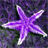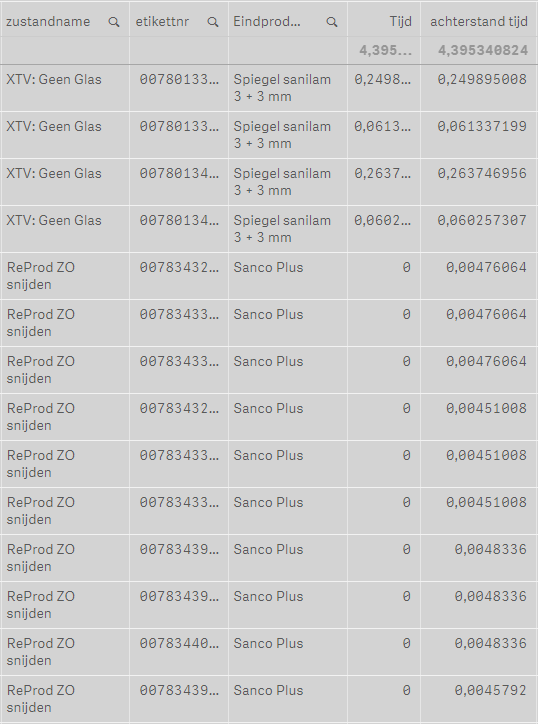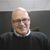# New to Qlik Sense

If you’re new to Qlik Sense, start with this Discussion Board and get up-to-speed quickly.

cancel
Showing results for
Did you mean:Contributor

## sum of column

hello,

I have a formula to make certain values 0 => if(Afdeling='Snijden',if(left(zustandname,6)='ReProd','0',Sum(([A.ZEIT]/A.STUECK)*(A.STUECK-A.FERTIG)))).

If I make the sum of this column he allso counts the values which are 0.

how can I solve this problem?THX

5 RepliesPartner - Champion III

The NULL will never be counted. The Count()-function will just skip the nulls and that goes for other aggregation functions as well along with the RangeXXX-functions too. In expressions outside of aggregations it will often prevent a numeric result.

The null can be produced by the Null() function and an If() function lacking a third parameter will return NULL if the If() function evaluates to false.

Maybe this will produce the right result for you:

if(Afdeling='Snijden',if(left(zustandname,6)='ReProd',Null(),Sum(([A.ZEIT]/A.STUECK)*(A.STUECK-A.FERTIG))))

Normally Afdeling and zustandname should also be wrapped in an aggregation function unless you are 100% sure that any of these fields never have more than one value at the row level in the table or chart you are using the expression in.Contributor III

Hi Kenny,

there are two ways to approach this - first is by substituting the 0 with null as suggested above, so that Qlik doesn't count it.

The other is to write the expression so that the Count evaluates to what you need in the total while returning the correct values in the column, that is, if you don't like the

You could take advantage of the dimensionality() function, which returns a 0 if the cell is a total, to return what you need and change the function.

=if(dimensionality() = 0,  [function to return what you need to count],   [function that returns what you need in the cells)

EricaPartner - Master III

let's try using the below expression:

=Sum( if( Afdeling = 'Snijden',

if( left(zustandname,6) = 'ReProd', 0, ( ([A.ZEIT]/A.STUECK)*(A.STUECK-A.FERTIG) ) ), 0

)

)Luminary Alumni

I think the problem here is with the Totals function for that column. Go to table properties, select the Tijd measure, and in the Total function dropdown select "Sum", which will do a sum of rows on the Totals rows.The reason why your expression returns a different result is that you expect is in the If statements and how does Qlik evaluate expressions on total rows. By default Qlik Sense evaluates the expression on total row exactly as on each individual row, only ignoring dimension fields. So in your case, Qlik Sense cannot determine which individual Afdeling or zustandname value you mean, because on total row there are many values coming to consideration. Therefore on Total row Afdeling='Snijden' evaluates to false, as well as left(zustandname,6) = 'ReProd'. Hence result of your expression.Contributor
Author

thank you allTags
Community Browser